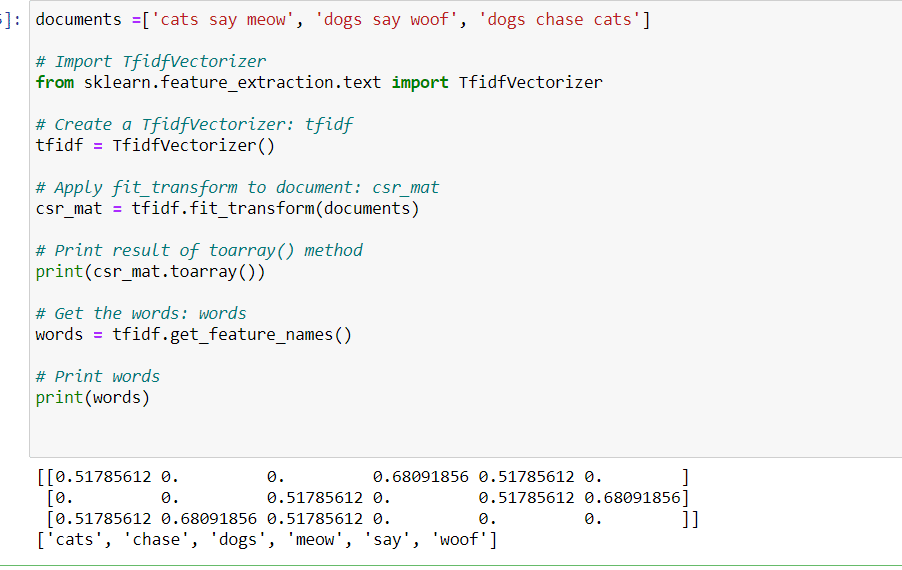# Dimension reduction with PCA

Dimension reduction represent the same data using less features and is vital for building machine learning pipelines using real-world data.

PCA performs dimension reduction by discarding the PCA features with lower variance, which it assumes to be noise, and retaining the higher variance PCA features, which it assumes to be informative.

To use PCA for dimension reduction, you need to specify how many PCA features to keep.  For example, specifying n_components=2 when creating a PCA model tells it to keep only the first two PCA features.  A good choice is the intrinsic dimension of the dataset, if you know it.

## Example

In a previous exercise, you saw that 2 was a reasonable choice for the “intrinsic dimension” of the fish measurements. Now use PCA for dimensionality reduction of the fish measurements, retaining only the 2 most important components.

The fish measurements have already been scaled for you, and are available as scaled_samples.

You can acces full code below link.

```from sklearn.decomposition import PCA

# Create a PCA model with 2 components: pca
pca = PCA(n_components=2)

# Fit the PCA instance to the scaled samples
pca.fit(scaled_samples)

# Transform the scaled samples: pca_features
pca_features = pca.transform(scaled_samples)

# Print the shape of pca_features
print(pca_features.shape)```

# Dimension reduction with PCA

### A tf-idf word-frequency array

In this exercise, you’ll create a tf-idf word frequency array for a toy collection of documents. For this, use the TfidfVectorizer from sklearn. It transforms a list of documents into a word frequency array, which it outputs as a csr_matrix. It has fit() and transform() methods like other sklearn objects.

```# Import TfidfVectorizer
from sklearn.feature_extraction.text import TfidfVectorizer

# Create a TfidfVectorizer: tfidf
tfidf = TfidfVectorizer()

# Apply fit_transform to document: csr_mat
csr_mat = tfidf.fit_transform(documents)

# Print result of toarray() method
print(csr_mat.toarray())

# Get the words: words
words = tfidf.get_feature_names()

# Print words
print(words)

```See you in the next article ..

10,081 views last month,  2 views today